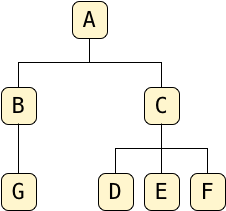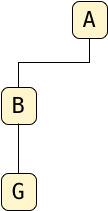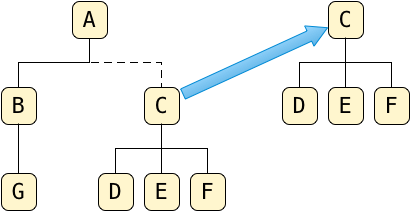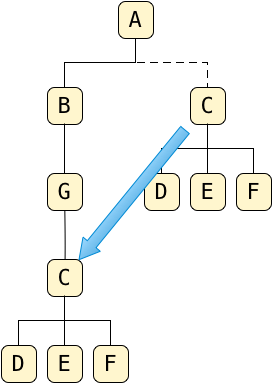# Manipulating Trees Using SQL and the Postgres LTREE Extension

### Today, I'll show you how to delete, move, and copy branches from one place to another in your tree using @> in combination with other LTREE functions.

· Database Zone · Tutorial
Save
14.37K Views

previously , i used the ltree extension to save a tree data structure in a postgres table. after saving the tree, i used the ``` @> ``` , or ancestor operator, to count the number of descendant nodes on a given branch.

but that's not all ltree can do. today, i'll show you how to delete, move, and copy branches from one place to another in your tree using ``` @> ``` in combination with other ltree functions. after that, in my last post in this series, i'll look at how ltree works under the hood and explore the computer science that makes all of this possible.

## my example tree again

here's the tree i've been working with during the last few blog posts:in my last post , i saved this tree in my database using a series of ``` insert ``` statements:

``````insert into tree (letter, path) values ('a', 'a');
insert into tree (letter, path) values ('b', 'a.b');
insert into tree (letter, path) values ('c', 'a.c');
insert into tree (letter, path) values ('d', 'a.c.d');
insert into tree (letter, path) values ('e', 'a.c.e');
insert into tree (letter, path) values ('f', 'a.c.f');
insert into tree (letter, path) values ('g', 'a.b.g');``````

and we saw how easy it is to count the number of tree nodes in a given branch using the ``` @> ``` operator:

``select count(*) from tree where 'a.c' @> path;``

## cutting off a branch

but suppose i wanted to remove these nodes from the tree entirely; that is, suppose i wanted to "cut off this branch" of the tree, so to speak:how can i do this? simple! i just use a sql ``` delete ``` statement:

``delete from tree where 'a.c' @> path;``

as you can see, i can use ``` @> ``` equally well in ``` delete ``` statements as in ``` select ``` statements.

## replanting a branch as a new tree

now suppose i want to keep this branch and save it as a separate tree in my table. that is, i want two trees: the original a tree and a new tree consisting of the c branch "replanted" as a new root:thinking about this for a moment, moving some nodes from one location to another in my tree means i'll need to update their path values somehow in my table; that is, i'll need to use an ``` update ``` statement and not a ``` select ``` or ``` delete ``` statement. but how? writing an ``` update ``` statement is easy enough, but how do i know what the new path of each tree node will be? let's take c as an example. because c will become the root node of my new tree, i want to change its path from a.c to just c:

``update tree set path = 'c' where path = 'a.c';``

and i will want to update d, one of c's children, in a similar way:

``update tree set path = 'c.d' where path = 'a.c.d';``

i could write a separate update statement for each node — just four sql statements for my example. but imagine i had hundreds or thousands of nodes in my tree. updating the records one sql statement at a time would require repeated network connections from my application to postgres, slowing down the overall operation tremendously.

instead, i need to update the path of c and each of its descendants all in a single operation. but how can i do this? two ltree functions, ``` nlevel() ``` and ``` subpath() ``` , can help.

## the nlevel function

first, ``` nlevel ``` . as you might guess, this returns the number of levels in a given path string:

``````select letter, path, nlevel(path) from tree;

letter | path  | nlevel
-------+-------+--------
a      | a     |      1
b      | a.b   |      2
c      | a.c   |      2
d      | a.c.d |      3
e      | a.c.e |      3
f      | a.c.f |      3
g      | a.b.g |      3
(7 rows)``````

looking at this, it's easy to understand what the function returns: for a root node like a, ``` nlevel ``` returns 1. for a's child nodes, a.b and a.c, ``` nlevel ``` returns 2. for the grandchild nodes, it returns 3. it simply counts the number of levels in each path string; internally, it parses the path string for period characters.

before we continue, consider one subtle but important point. notice that i was able to calculate ``` nlevel ``` on all of the records in the tree table with a single sql statement! postgres applied the function to all of the matching paths for me. the power of ltree's functions is that they seamlessly integrate with sql, harnessing and extending the power of postgres.

## the subpath function

ltree provides another new sql function that will also help us write a general tree path formula: ``` subpath ``` . as you might guess, this returns a selected substring from a given path. let's try running it on my example tree:

``````select letter, subpath(path, 1) from tree;
error:  invalid positions
statement:  select letter, subpath(path, 1) from tree;``````

oops — i've done something wrong here. calling ``` subpath(path, 1) ``` returns the portion of the path starting with offset 1. not a character offset, but a path offset . so ``` subpath(path, 1) ``` drops the first level of the path, a in my tree, and returns the remaining portion of each path starting from the second path element. internally, ltree parses the periods for me, drops the requested number of path levels, and removes the extra leading period.

in the statement above, the error was caused by the root node in the tree: a. this path has only one level, and so ltree returns an error in this case.

let's try using ``` subpath ``` only on the c branch — the branch we want to move:

``````select letter, subpath(path, 1) from tree where path <@ 'a.c';
letter | subpath
-------+---------
c      | c
d      | c.d
e      | c.e
f      | c.f
(4 rows)``````

now, i get only four records in the result: one for c and one for each node that appears under c. and you can see that the ``` subpath ``` column contains the portion of the path that appears after a, for each of these four records.

and again, notice that i was able to execute the ``` subpath ``` function on all four tree records that i wanted to — in a single operation. this time, the ``` subpath ``` function worked in concert with the ``` <@ ``` operator. ltree has made the sql language i already know how to use even more powerful.

## moving tree nodes using one update statement

now, let’s return to the question of moving a branch into a new tree. as this diagram shows, i want to delete c and its children from the a tree and move them to a new location:earlier, i considered moving the nodes using a single ``` update ``` statement for each:

``````update tree set path = 'c' where path = 'a.c';
update tree set path = 'c.d' where path = 'a.c.d';
update tree set path = 'c.e' where path = 'a.c.e';
update tree set path = 'c.f' where path = 'a.c.f';``````

now that i know about ``` subpath ``` , it’s easy to write a single sql update statement that will move all four nodes in the c branch in one operation:

``update tree set path = subpath(path, 1) where path <@ 'a.c';``

i use ``` where path <@ 'a.c' ``` to scope the update to the c branch and i use ``` subpath(path, 1) ``` to remove the a root element from the path of c and each of its descendants.

i can generalize this a bit more using the ``` nlevel ``` function, also:

``update tree set path = subpath(path, nlevel('a.c')-1) where path <@ 'a.c';``

this follows because ``` nlevel('a.c') = 2 ``` , and therefore, ``` nlevel('a.c')-1 ``` returns the same formula we had above. replacing a.c with ``` branch_path ``` , i arrive at a general formula for “replanting” a branch as a new tree using a single sql statement:

``update tree set path = subpath(path, nlevel(branch_path)-1) where path <@ branch_path``

…assuming ``` nlevel(branch_path) > 1 ``` , that is assuming the branch we want to replant isn’t already a root.

## the || concatenation operator

this seems somewhat useful, but what if i want to move a branch from one location in my tree to some other location, not necessary to the root? this is a more general problem. for example, suppose i want to move the c branch under g, like this:to write a formula for this transformation using sql, we need to use one more important ltree operator: the ``` || ``` or concatenation operator. let’s try it out with an example first:

``````select 'a.b.g' || path as concatenated from tree;
concatenated
--------------
a.b.g.a
a.b.g.a.b
a.b.g.a.c
a.b.g.a.c.d
a.b.g.a.c.e
a.b.g.a.c.f
a.b.g.a.b.g
(7 rows)``````

you can see ltree has automatically added ``` a.b.g ``` , along with a period separator to each path in my table. and it has done this for all the paths in my table in a single operation.

## moving a branch

now, using ``` || ``` , i can write a single sql statement to move a tree branch from one location to another. first, of course, i need to scope the sql operation to the target branch using the ancestor operator:

``````select 'a.b.g' || path as concatenated from tree where path <@ 'a.c';
concatenated
---------------
a.b.g.a.c
a.b.g.a.c.d
a.b.g.a.c.e
a.b.g.a.c.f
(4 rows)``````

i get the same results as above, but now only for the tree nodes that i want to move.

but my next problem is the new paths above start with ``` a.b.g.a.c… ``` . instead, i want them to be ``` a.b.g.c… ``` . i need to remove that extra a character from the new paths, using the ``` subpath ``` operator:

``````select 'a.b.g' || subpath(path, 1) as concatenated from tree where path <@ 'a.c';
concatenated
--------------
a.b.g.c
a.b.g.c.d
a.b.g.c.e
a.b.g.c.f
(4 rows)``````

and finally, converting this into an update statement:

``````update tree set path = 'a.b.g' || subpath(path, 1) where path <@ 'a.c'
``````

…i have the single sql statement i need!

and generalizing this, we arrive at a sql formula you could use in your own postgres database:

``````update tree set path = destination_path || subpath(path, nlevel(source_path)-1)
where path <@ source_path;``````

## copying a branch

one last puzzle: how can i copy a tree branch instead of moving it? i just use an insert sql statement instead of update. simple, right?

but how, exactly? i need to insert multiple rows — one record for each node in the branch i copy. again, i could write a series of insert statements like this:

``````insert into tree (letter, path) values ('c', 'a.b.g.c');
insert into tree (letter, path) values ('d', 'a.b.g.c.d');
insert into tree (letter, path) values ('e', 'a.b.g.c.e');
insert into tree (letter, path) values ('f', 'a.b.g.c.f');``````

but using ltree functions and operators, i can achieve this using a single sql statement! i just have to write sql that will insert the result of a select, like this:

``````insert into tree (letter, path) (
select letter, 'a.b.g' || subpath(path, 1) from tree where 'a.c' @> path
)``````

executing this, postgres will first find all the nodes inside the branch i want to copy and recalculate their paths. then, it will insert that result set into the tree as a copy, leaving my original branch unchanged!

by writing this tree-related logic using ltree operators in sql, i ask postgres to do all of the hard work of manipulating and copying the path strings for me. i don’t have to write application code to keep track of these strings, and no data needs to be transmitted back and forth between my application server and the database server.

## what’s next? ltree internals

in my last post about ltree, i’ll look closely at how it works internally. it’s easy enough to imagine how simple functions like ``` nlevel ``` , ``` || ``` , or ``` subpath ``` work. that’s not the interesting part for me. these functions are shorthand for fairly simple string operations.

the special sauce that makes ltree such a powerful tool is that it integrates with postgres gist indexes. by using an index, postgres can execute any of the sql expressions i wrote above equally fast on 7,000 records as it would on 7! how? the only way to find out is by looking inside postgres at a gist index.

Topics:
database, tutorial, ltree, sql, postgres, nlevel, subpath, concatenation

Published at DZone with permission of Pat Shaughnessy, DZone MVB.

Opinions expressed by DZone contributors are their own.